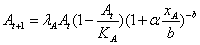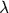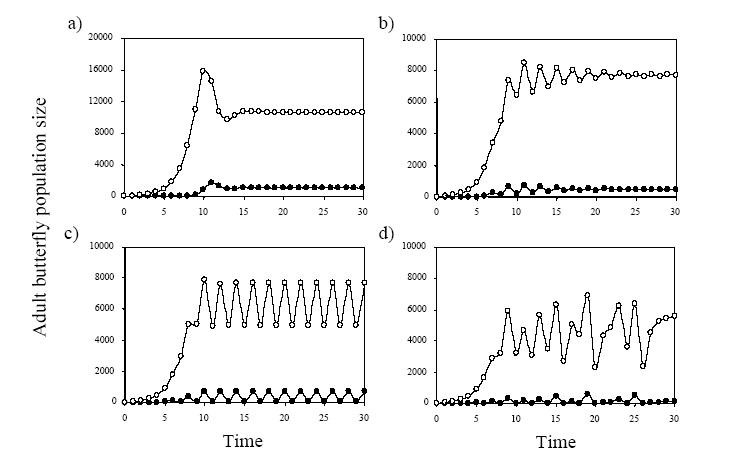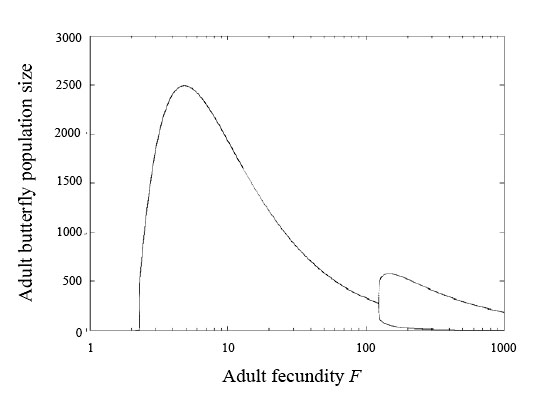### Nicolas Mouquet, Jeremy A. Thomas, Graham W. Elmes, Ralph T. Clarke, and Michael E. Hochberg. 2005. Population dynamics and conservation of a specialized predator: a case study of Maculinea arion. Ecological Monographs 75:525–542.

Appendix D. Population dynamics of ants.

Here we briefly explore the consequences of explicit ant nest dynamics. The host ants can have complex dynamics associated with environmental parameters (see Hochberg et al. 1994, Clarke et al. 1997 ). Here, we propose a simpler model with ant nest growth and death rates directly linked to the population dynamics of the butterfly and to intraspecific competition. The equation describing ant nest changes is:(D.1)

The equation resembles classical models of host-parasitoid interactions in which the host is limited by intraspecific competition in the absence of the parasitoid (e.g., May et al. 1981, Hochberg and Lawton 1990 ). We assume that the two forms of density dependence act independently. The basic growth rate of the ant nest population isA . The first term in brackets describes  intraspecific competition, with KA being the ant   population size above which the effect of intraspecific competition becomes marked. The second term in brackets describes the mortality due to caterpillar parasitism. As previously assumed, the distribution of caterpillars among ant nests follows a negative binomial distribution (more aggregated than random) with clumping parameter k (b = k + 1). a is a scaling parameter.

Figure D1 shows examples of the dynamics for different parameter combinations. A familiar result of such models is that host populations (i.e., the ants) first increase exponentially from rarity until they are controlled by the enemy (i.e., the caterpillars) (Fig. D1a). For higher population growth rates and more random caterpillar distributions (high b), more complex dynamics are obtained, ranging from oscillatory damping (Fig. D1b) to cycles (Fig. D1c) and chaos (Fig. D1d). The same potential for complex dynamics therefore exists with and without explicit changes in the ant population, but we find that the limit to complex dynamics is shifted to higher values of species parameters.FIG. D1. Example of time series obtained for M. arion (empty circles) and M. sabuleti (black circles) for the model described by equations (9) and (D1). We have varied species fecundity F and the density dependence parameter within ant nests b to generate four different dynamics. (a) Oscillatory damping (F = 25.5, b = 2) and (b) (F = 65, b = 20), (c) cycles (F = 100 b = 20) and (d) chaos (F = 350, b = 50). We choseA , a and KA  so that the equilibrium value of the ants nest was equal to what would have been used in a model without explicit ant nest dynamics (A » 10000):A = 2, KA = 50000 and= 0.00005. Other parameters as in scenario #1 (Table 2).

For example, Fig. D2 shows the bifurcation diagram obtained with varying butterfly fecundity. In comparison to Fig. 4b (generated with the same set of parameters but without the ant dynamics), the onset of cycles is shifted to higher fecundity values. This is due to the coupling between the dynamics of M. arion and the ant nests, which acts as a buffer against overcompensation and thus stabilizes the butterfly’s population compared to when ant nest numbers are set constant. This suggests that systems are more stable when one considers explicit ant nest dynamics.FIG. D2. Bifurcation diagram of M. arion butterfly adult population size with varying species adult fecundity F, obtained with the model described in Eqs. 9 and 20. Other parameters as in scenario #1 (Table 2), except b = 10.

LITERATURE CITED

Clarke, R. T., J. A. Thomas, G. W. Elmes, and M. E. Hochberg. 1997. The effect of spatial patterns in habitat quality on community dynamics within a site. Proceedings of the Royal Society of London Serie B 264:347–354.

Hochberg, M. E., R. T. Clarke, G. W. Elmes, and J. A. Thomas. 1994. Population-dynamic consequences of direct and indirect interactions involving a large blue butterfly and its plant and red ant hosts. Journal of animal ecology 63:375–391.

Hochberg, M. E., and J. H. Lawton. 1990. Spatial heterogeneity in parasitism and population dynamics. Oikos 59:9–14.

May, R. M., M. P. Hassell, R. M. Anderson, and D. W. Tonkyn. 1981. Density dependence in host-parasitoid models. Journal of animal ecology 50:855–865.

[Back to M075-021]